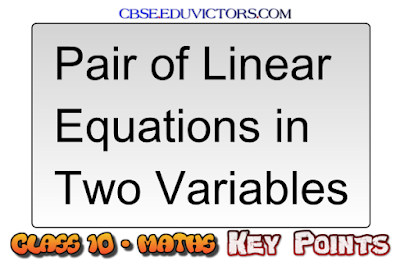Pair of Linear Equations in Two Variables - Key Points

Linear Equation

An equation which can be put in the form ax + by + c = 0, where a, b and c are real numbers and both a and b are nonzero is called a linear equation in two variables.

The solution of an Equation
Each solution (x, y) of a linear equation in two variables.

ax + by + c = 0, corresponds to a point on the line representing the equation, and vice-versa.

Pair of Linear Equations in Two Variables

The general form for a pair of linear equations in two variables x and y is
a₁x + b₁y + c₁ = 0
and a₂x + b₂y + c₂ = 0

Geometrically they look like the following:

Graphical Method of Solutions

x – 2y = 0

3x + 4y = 20

The graph  drawn is:

The solution is (4, 2), the point of intersection.

To summarize the behaviour of lines representing a pair of linear equations in two variables:

- The lines may intersect in a single point. In this case, the pair of equations has a unique solution (consistent pair of equations).

- The lines may be parallel. In this case, the equations have no solution (inconsistent pair of equations).

- The lines may be coincident. In this case, the equations have infinitely many solutions [dependent (consistent) pair of equations]

Substitution Method

The following are the steps:

Find the value of one variable, say y in terms of the other variable, i.e., x from either equation, whichever is convenient.

Substitute this value of y in the other equation, and reduce it to an equation in one variable, i.e., in terms of x, which can be solved. Sometimes, you can get statements with no variable.

If this statement is true, you can conclude that the pair of linear equations has infinitely many solutions. If the statement is false, then the pair of linear equations is inconsistent.

Substitute the value of x (or y) obtained in Step 2 in the equation used in Step 1 to obtain the value of the other variable.

Elimination Method

Steps in the elimination method:
First, multiply both the equations by some suitable non-zero constants to make the coefficients of one variable (either x or y) numerically equal.

Then add or subtract one equation from the other so that one variable gets eliminated. If you get an equation in one variable, go to Step 3.

If in Step 2, we obtain a true statement involving no variable, then the original pair of equations has infinitely many solutions.
If in Step 2, we obtain a false statement involving no variable, then the original pair of equations has no solution, i.e., it is inconsistent.

Solve the equation in one variable (x or y) so obtained to get its value.

Substitute this value of x (or y) in either of the original equations to get the value of the other variable.

Cross Multiplication Method
Steps:
Write the given equations in the form

a₁x + b₁y + c₁ = 0
and a₂x + b₂y + c₂ = 0

Taking the help of the diagram

Write Equations as

Find x and y, provided a₁b₂ -  a₂b₁ ≠ 0

CH 1: Real Numbers (MCQ)
CH 1: Real  Numbers (Study Points)
CH 1: Real Numbers (NCERT Ex 1.1)
CH 1: Real Numbers (Euclid's Division Lemma - Q & A)
CH 1: Problems on Euclid's Division Algorithm
CH 1: Real Numbers (Problems and Answers)
CH 1: Real Numbers (NCERT Ex 1.2)
CH 1: Real Numbers (NCERT Exemplar Ex 1.1 Q1-3)
CH 1: Real Numbers (NCERT Exemplar Ex 1.1 Q4-6)

CH 2: Polynomials (Study Points)
CH 2: Polynomials (NCERT Ex 2.1)
CH 2: Polynomials (NCERT Ex 2.2)

CH 3: Linear Equations In Two Variables (Revision Assignment)
CH 3: Linear Equations In Two Variables (Solved Examples)
CH 3: Linear Equations In Two Variables (Key Points)

CH 4 : Quadratic Equations (Ex 4.1)
CH 4 : Quadratic Equations (NCERT Ex 4.2)

CH 5: Arithmetic Progressions (MCQs)
CH5: Arithmetic Progressions - Seven Problems You Must Know
CH5: Arithmetic Progressions (Q & A)

CH 6: Triangles (Important Points To Remember)
Lines and Angles (Brain Teaser)

CH 8: Introduction to Trigonometry (MCQs from CBSE Papers)
CH 8: Introduction to Trigonometry (NCERT Ex 8.1)
CH 8: Introduction to Trigonometry (NCERT Ex 8.2)

CH 8: Problems based on Trigonometric Identities
CH 8: Problems based on Trigonometric Identities (Set-2)
CH 8: Introduction to Trigonometry (Short Q & A)

CH 9: Applications of Trigonometry (MCQs) or Online Quiz
CH 14: Statistics (NCERT Ex 14.1)

CH 15: 5 Minutes Revision on Probability
CH 15: 5 Questions on Probability Your Teacher Can Ask To Confuse You